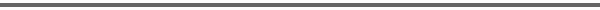Journal Home Page Cumulative Index List of all Volumes Complete Contentsof this Volume Previous Article Next Article Journal of Convex Analysis 11 (2004), No. 2, 335--361 Copyright Heldermann Verlag 2004Variational Analysis for a Class of Minimal Time Functions in Hilbert Spaces Giovanni Colombo Dip. di Matematica Pura e Applicata, Universita di Padova, Via Belzoni 7, 35131 Padova, Italy, colombo@math.unipd.it Peter R. Wolenski Dept. of Mathematics, Louisiana State University, 326 Lockett Hall, Baton Rouge, LA 70803-4918, U.S.A., wolenski@math.lsu.edu[Abstract-pdf] \def\iint{{\hbox{int}\;}} This paper considers the parameterized infinite dimensional optimization problem $$\hbox{minimize}\quad\bigl\{t\geq 0:\;S \cap\{x+tF\}\not= \emptyset\bigr\},$$ where $S$ is a nonempty closed subset of a Hilbert space $H$ and $F\subseteq H$ is closed convex satisfying $0\in \iint F$. The optimal value $T(x)$ depends on the parameter $x\in H$, and the (possibly empty) set $S\cap (x+T(x)F)$ of optimal solutions is the $F$-projection'' of $x$ into $S$. We first compute proximal and Fr\'echet subgradients of $T(\cdot)$ in terms of normal vectors to level sets, and secondly, in terms of the $F$-projection. Sufficient conditions are also obtained for the differentiability and semiconvexity of $T(\cdot)$, results which extend the known case when $F$ is the unit ball. FullText-pdf (641 KB) for subscribers only.